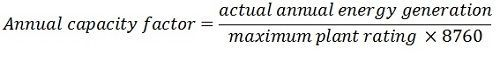# Capacity Factor

The capacity factor is defined as the ratio of the total actual energy produced or supply over a definite period, to the energy that would have been produced if the plant (generating unit) had operated continuously at the maximum rating. The capacity factor mainly depends on the type of the fuel used in the circuit.The capacity factor is computed by dividing the total energy producing by the full load capacity of the plant. Capacity factor is mostly used in generation studies. The annual capacity factor is expressed asCapacity factor indicates the extent of the use of the generating station. If the power generation unit is always running at its rated capacity, then their capacity factor is 100% or 1. It is also expressed regarding peak load and load factor.The power plant always has some reserve capacity for the future expansion like an increase in load and maintenance. If the rate plant capacity is equal to the peak load, then the capacity factor and load factor become identical, i.e. in the absence of reverse capacity.